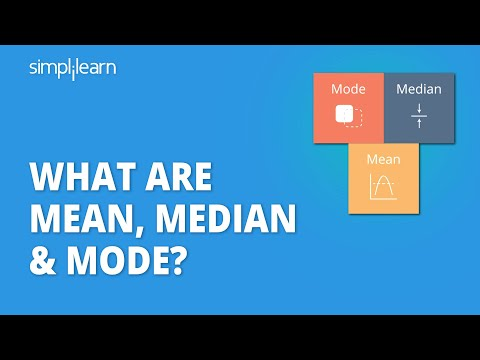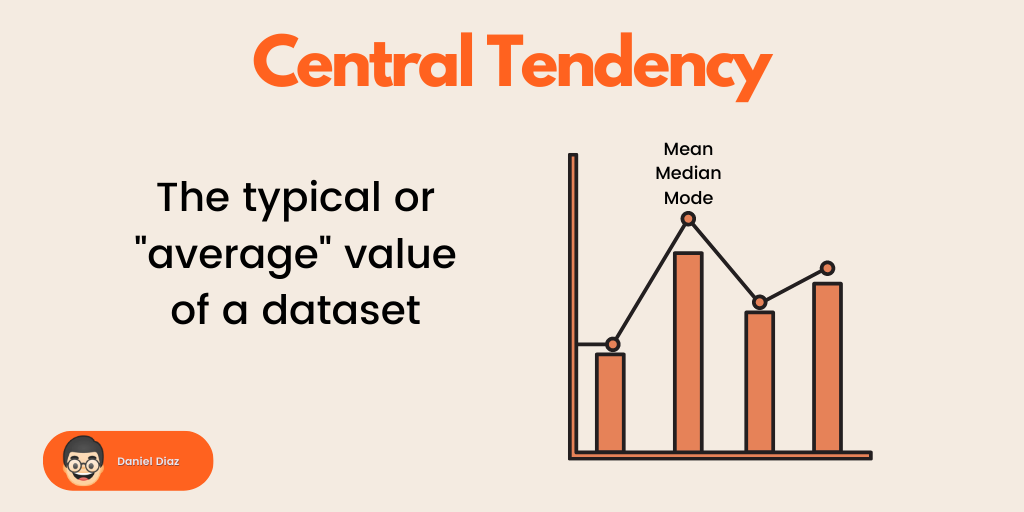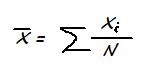1623052997

# What Are Mean, Median & Mode? | Learn Statistics | Statistics Basic Concepts Tutorial

What are Mean, Median and Mode? Let’s Learn Statistics by answering these questions along with formulae and worked example to really understand the foundational concepts in this Statistics Basic Concepts Tutorial in depth. We will also take a look at geometric and harmonic mean, two sub-topics of arithematic mean. The topics covered in this video are :

• 00:00 What is Arithematic Mean?
• 03:21 What is Median?
• 06:01 What is Mode?
• 09:11 What is Geometric Mean?
• 10:41 What is Harmonic Mean?

What is Mean, Median and Mode?

Mean the the sum average of all data values and gives us the value which is most common in our data and around which the rest of our data values lie.
The median is the middle score for a set of data that has been arranged in order of magnitude. The median is less affected by outliers and skewed data and is more reliable than mean.
The mode is the most frequently occuring value in our data. On a histogram it represents the highest bar in a bar chart or histogram. You can, therefore, sometimes consider the mode as being the most popular option.

#developer

## Buddha Community1624608788

## How to Find Mean, Median, and Mode in Python?

Mean, median, and mode are fundamental topics of statistics. You can easily calculate them in Python, with and without the use of external libraries.

These three are the main measures of central tendency. The central tendency lets us know the “normal” or “average” values of a dataset. If you’re just starting with data science, this is the right tutorial for you.By the end of this tutorial you’ll:

• Understand the concept of mean, median, and mode
• Be able to create your own mean, median, and mode functions in Python
• Make use of Python’s statistics module to quickstart the use of these measurements

If you want a downloadable version of the following exercises, feel free to check out the GitHub repository.

Let’s get into the different ways to calculate mean, median, and mode.

#development #python #how to find mean, median, and mode in python #find mean #median #mode1595334123

## Authentication In MEAN Stack - A Quick GuideI consider myself an active StackOverflow user, despite my activity tends to vary depending on my daily workload. I enjoy answering questions with angular tag and I always try to create some working example to prove correctness of my answers.

To create angular demo I usually use either plunker or stackblitz or even jsfiddle. I like all of them but when I run into some errors I want to have a little bit more usable tool to undestand what’s going on.

Many people who ask questions on stackoverflow don’t want to isolate the problem and prepare minimal reproduction so they usually post all code to their questions on SO. They also tend to be not accurate and make a lot of mistakes in template syntax. To not waste a lot of time investigating where the error comes from I tried to create a tool that will help me to quickly find what causes the problem.

``````Angular demo runner
Online angular editor for building demo.
ng-run.com
<>
``````

Let me show what I mean…### Template parser errors#

There are template parser errors that can be easy catched by stackblitzIt gives me some information but I want the error to be highlighted#mean stack #angular 6 passport authentication #authentication in mean stack #full stack authentication #mean stack example application #mean stack login and registration angular 8 #mean stack login and registration angular 9 #mean stack tutorial #mean stack tutorial 2019 #passport.js1574339995

## Learn Python Tutorial from Basic to Advance

Description
Become a Python Programmer and learn one of employer’s most requested skills of 21st century!

This is the most comprehensive, yet straight-forward, course for the Python programming language on Simpliv! Whether you have never programmed before, already know basic syntax, or want to learn about the advanced features of Python, this course is for you! In this course we will teach you Python 3. (Note, we also provide older Python 2 notes in case you need them)

With over 40 lectures and more than 3 hours of video this comprehensive course leaves no stone unturned! This course includes tests, and homework assignments as well as 3 major projects to create a Python project portfolio!

This course will teach you Python in a practical manner, with every lecture comes a full coding screencast and a corresponding code notebook! Learn in whatever manner is best for you!

We will start by helping you get Python installed on your computer, regardless of your operating system, whether its Linux, MacOS, or Windows, we’ve got you covered!

We cover a wide variety of topics, including:

Command Line Basics
Installing Python
Running Python Code
Strings
Lists
Dictionaries
Tuples
Sets
Number Data Types
Print Formatting
Functions
Scope
Built-in Functions
Debugging and Error Handling
Modules
External Modules
Object Oriented Programming
Inheritance
Polymorphism
File I/O
Web scrapping
Database Connection
Email sending
and much more!
Project that we will complete:

Guess the number
Guess the word using speech recognition
Love Calculator
Click and save image using openCV
Ludo game dice simulator
open wikipedia on command prompt

So what are you waiting for? Learn Python in a way that will advance your career and increase your knowledge, all in a fun and practical way!

Basic knowledge
Basic programming concept in any language will help but not require to attend this tutorial
What will you learn
Learn to use Python professionally, learning both Python 2 and Python 3!
Create games with Python, like Tic Tac Toe and Blackjack!
Learn advanced Python features, like the collections module and how to work with timestamps!
Learn to use Object Oriented Programming with classes!
Understand complex topics, like decorators.
Understand how to use both the pycharm and create .py files
Get an understanding of how to create GUIs in the pycharm!
Build a complete understanding of Python from the ground up!

#Learn Python #Learn Python from Basic #Python from Basic to Advance #Python from Basic to Advance with Projects #Learn Python from Basic to Advance with Projects in a day1597449780

## Introduction

In statistics, measures of central tendency are a set of “middle” values representative of the data points. Central tendency describes the distribution of data focusing on the central location around which all other data are clustered. It is the opposite of dispersion that measures how far the observations are scattered with respect to the central value.

As we will see below, central tendency is an elementary statistical concept, yet a widely used one. Among the measures of central tendency mean, median and mode are most frequently cited and used. Below we will see why they are important in the field of data science and analytics.

## 1. Arithmetic Mean

Mean is the average of some data points. It is the simplest measure of central tendency that takes the sum of the observations and divides the sum by the number of observations.

In mathematical notation arithmetic mean is expressed as:Where xi are individual observations and N is the number of observations

In a more practical example, if wages of 3 restaurant employees are \$12, \$14 and \$15 per hour, then the average wage is \$13.6 per hour. Simple as that.

#statistics #machine-learning #median #data-science #mean #deep learning1625992020

## Mean, Median and Mode with JavaScript

In this video, we are implementing algorithms for calculating the mean, median and mode of a dataset with JavaScript.

Before proceeding to coding, we briefly discuss how we can calculate each of these dataset properties.

Mean: in order to calculate the mean, all we have to do is to sum up all data points and divide by the number of data points. In other words, the mean, and more specifically the arithmetic mean (which is what we are calculating today), is what we usually call “the average” in everyday-life. Although, technically, mean, median, and mode are all kinds of averages, each trying to summarize the dataset with a single number representing a “typical” data point from the dataset.

Median: in order to get the median (the middle value), we first need to sort the dataset (conventionally in ascending order, but we would get the same result by sorting in descending order) and pick the data-point in the middle. If we had an even number of data points, we would get the mean of the 2 middle elements (by adding them and dividing by 2).

Mode: is the value in the dataset which occurs more frequently. If all values in the dataset appear with the same frequency, then the dataset has no mode. It is also possible for a dataset to have more than one modes.

Mean, median and mode, are different approaches for calculating the “typical” or “central” value of a dataset. That’s why they are also called measures of central tendency. Usually, they are used in conjunction with measures of spread (or measures of dispersion), in order to get the degree to which data is distributed around the center. Such measures of spread are the Range (which is the difference between the maximum and minimum value of the dataset) and Variance (or Standard Deviation which is the square root of Variance).

In this video, we are implementing algorithms for measures of central tendency. Feel free to expand on this, maybe by also calculating measures of spread and/or other statistical values. You could even take it a step further by creating a user interface such as a statistics calculator, which allows the user to enter a dataset and calculates and presents to the user a number of statistical values for the given dataset.

00:00 - Introduction to Concepts
04:15 - Implementation of Algorithms

Enjoy 😊

Don’t forget to like, share, subscribe and I would love to hear your thoughts in the comments section below!

Code for this Project: https://codepen.io/Coding_Journey/pen/VweybWG

Support the Channel 💙☕🙏
PayPal: https://paypal.me/CodingJourney